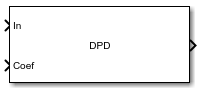DPD

Digital predistorter

• Library:
• Communications Toolbox / RF Impairments Correction

•Description

Apply digital predistortion (DPD) to a complex baseband signal using a memory polynomial to compensate for nonlinearities in a power amplifier. For more information, see Digital Predistortion.

This icon shows the block with all ports enabled.Ports

Input

expand all

Input baseband signal, specified as a column vector. This port is unnamed until the Coefficient source parameter is set to Input port.

Data Types: double
Complex Number Support: Yes

Memory-polynomial coefficients, specified as a matrix. The number of rows in the matrix must equal the memory depth of the memory polynomial.

• If the Polynomial type parameter is set to Memory polynomial, the number of columns in the matrix is the degree of the memory polynomial.

• If Polynomial type is set to Cross-term memory polynomial, the number of columns in the matrix must equal m(n-1)+1. m is the memory depth of the polynomial, and n is the degree of the memory polynomial.

Example: complex([1 0 0 0 0; 0 0 0 0 0; 0 0 0 0 0])

Dependencies

To enable this port, set the Coefficient source parameter to Input port.

Data Types: double
Complex Number Support: Yes

Output

expand all

Predistorted baseband signal, returned as a column vector of the same length as the input signal.

Parameters

expand all

Polynomial type used for predistortion, specified as one of these values:

• Memory polynomial — Computes predistortion coefficients by using a memory polynomial without cross terms

• Cross-term memory polynomial — Computes predistortion coefficients by using a memory polynomial with cross terms

Source of the memory polynomial coefficients, specified as one of these values:

• Property — Specify this value to use the Coefficients parameter to define the memory-polynomial coefficients

• Input port — Specify this value to use the Coef input port to define the memory-polynomial coefficients

Memory-polynomial coefficients, specified as a matrix. The number of rows must equal the memory depth of the memory polynomial.

• If the Polynomial type is set to Memory polynomial, the number of columns is the degree of the memory polynomial.

• If the Polynomial type is set to Cross-term memory polynomial, the number of columns must equal m(n-1)+1. m is the memory depth of the polynomial, and n is the degree of the memory polynomial.

Dependencies

To enable this parameter, set Coefficient source to Property.

Data Types: double
Complex Number Support: Yes

Type of simulation to run, specified as Code generation or Interpreted execution.

• Code generation –– Simulate the model by using generated C code. The first time you run a simulation, Simulink® generates C code for the block. The C code is reused for subsequent simulations unless the model changes. This option requires additional startup time, but the speed of the subsequent simulations is faster than Interpreted execution.

• Interpreted execution –– Simulate the model by using the MATLAB® interpreter. This option requires less startup time than the Code generation method, but the speed of subsequent simulations is slower. In this mode, you can debug the source code of the block.

Block Characteristics

 Data Types double | single Multidimensional Signals no Variable-Size Signals yes